If there are 7 apples and 5 oranges in the basket then what fraction of oranges are there in the fruit basket?

o =  0.4167

### Step-by-step explanation:

$o=\frac{5}{7+5}=\frac{5}{12}=0.4167$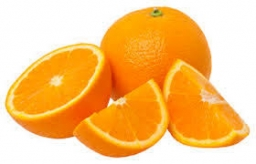Did you find an error or inaccuracy? Feel free to write us. Thank you!Tips to related online calculators
Need help to calculate sum, simplify or multiply fractions? Try our fraction calculator.

## Related math problems and questions:The weight of the empty fruit basket is ¾ kg. We added ½ kg apples and 5/4 kg guavas. What will be the total weight of the fruit basket?In six baskets, the seller has fruit. In individual baskets, there are only apples or just pears with the following number of fruits: 5,6,12,14,23 and 29. "If I sell this basket," the salesman thinks, "then I will have just as many apples as a pear." WhicThe fruit basket is five times heavier than the empty basket. Filled is 20kg heavier than empty. Determine the weight of the fruit in the basket.
• Chocolate to sweetsI have 30 basket sweets and 15 chocolate in a basket. If take 5 sweet out of the basket what is the ratio in simplest form of the chocolate to the numbers of sweet left in the basket?
• The cost 2The cost of 5 apples is $3.45 and 5 oranges is$1.23. If Rachel buys one apple and one orange, then how much must she pay?
• FruitsAmy bought a basket of fruits 1/5 of them were apples,1/4 were oranges, and the rest were 33 bananas. How many fruits did she buy in all?
• There 13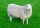There are 7 cows 3 sheeps and 4 chickens on a field . What fraction of the animals are sheep?
• Fruits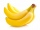For the price of one pineapple, I will buy two oranges. For the price of three oranges, I will buy four apples. I will buy 6 bananas for the price of three apples. What is the price of one banana if I pay 1 euro for one pineapple?
• Division by zero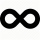Fraction 5 by 2. if 3 is added to numerator and 2 is subtracted from the denominator then the new fraction is:
• Children 9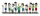There are 11 children in a room. 6 of the children are girls. What fraction of the children are girls?
• An orchardDuring a visit to an orchard, Greg picked 3/5 of a bag of delicious golden apples, 4/5 of a bag of Macintosh apples, 2/5 of a bag of Cortland apples, 1/5 of a bag of Bartlett pears, and 4/5 of a bag of Bosch pears. How many bags of fruit to Greg pick in t
• Denominator 2Denominator of a fraction is 5 and numerator is 7. Write the fraction .
• Fruit punchKelly made fruit punch to serve at a party for her chess team. She mixed 1 2/5  liters of cranberry juice and 1 3/5  liters of pineapple juice together. Then, she split the fruit punch evenly among 9 glasses. How much fruit punch did Kelly pour into each
• SchoolThere are 150 pupils in grade 5 . 2/3 of them are female. By what fractions are the males?
• WithdrawalIf I withdrew 2/5 of my total savings and spent 7/10 of that amount. What fraction do I have in left in my savings?
• Report 2A School reports students to teacher ratio of 6:1. If there are 45 teachers in the School, how many students are there?
• TreesFrom the total number of trees in the orchard, there are two-fifths pearls and apples are three eighty. The rest of the trees are 9 ceremonial. How many trees are in the set?